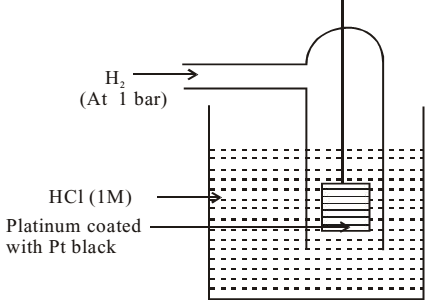# Measurement of Electrode Potential

It is not possible to measure single electrode potential. It is because the reaction taking place at the electrodes is oxidation or reduction and these reactions do not take place in isolation. It can be measured with respect to a reference electrode. The electrode used as reference electrode is standard hydrogen electrode (SHE).

### Standard Hydrogen Electrode

Standard Hydrogen Electrode (SHE) consists of a container, containing 1M HCl solution kept at 298 K. A wire containing platinum electrode coated with platinum black is immersed in the solution. Pure hydrogen gas is bubbled in the solution at 1 bar pressure.The potential of SHE (Eº) is taken as zero volt at all temperatures.

Standard hydrogen electrode may act as anode or cathode depending upon the nature of the other electrode. If its acts as anode, the oxidation reaction takes place.

H2 (g) → 2H+ (aq) + 2e

If it acts as cathode then the reduction half reaction occurs.

2H+ (aq) + 2e → H2 (g)

### Measurement of Standard Electrode Potential

Magnitude

The standard electrode potential of an electrode can be measured by combining it with standard hydrogen electrode. For example, a zinc strip is dipped in 1M ZnSO4 solution and it is connected to Standard Hydrogen electrode. The cell emf is found to be 0.76 V. When copper electrode i.e. copper dipped in 1M CuSO4 solution is connected to standard hydrogen electrode then the cell emf is 0.34 V.

Sign

The galvanic cell formed by the combination of SHE and electrode under study, the polarity of the electrode is determined with the help of a voltmeter. In case the given electrode is found to be positive electrode, its electrode potential is given the positive sign and if it is negative then it is given the negative sign. In the case of zinc connected to SHE the polarity is negative but in case of copper it is positive.